# Truncated dodecahedron

Truncated dodecahedron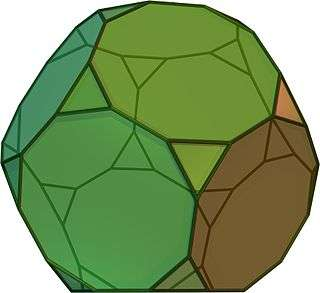TypeArchimedean solid
Uniform polyhedron
ElementsF = 32, E = 90, V = 60 (χ = 2)
Faces by sides20{3}+12{10}
Conway notationtD
Schläfli symbolst{5,3}
t0,1{5,3}
Wythoff symbol2 3 | 5
Coxeter diagramSymmetry groupIh, H3, [5,3], (*532), order 120
Rotation groupI, [5,3]+, (532), order 60
Dihedral Angle10-10: 116.57°
3-10: 142.62°
ReferencesU26, C29, W10
PropertiesSemiregular convex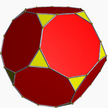Colored faces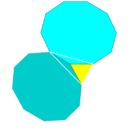3.10.10
(Vertex figure)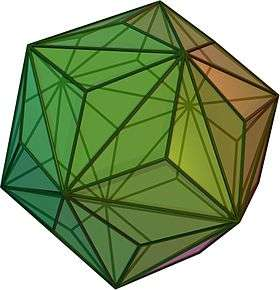Triakis icosahedron
(dual polyhedron)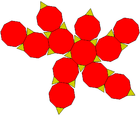Net

In geometry, the truncated dodecahedron is an Archimedean solid. It has 12 regular decagonal faces, 20 regular triangular faces, 60 vertices and 90 edges.

## Geometric relations

This polyhedron can be formed from a dodecahedron by truncating (cutting off) the corners so the pentagon faces become decagons and the corners become triangles.

It is used in the cell-transitive hyperbolic space-filling tessellation, the bitruncated icosahedral honeycomb.

## Area and volume

The area A and the volume V of a truncated dodecahedron of edge length a are:## Cartesian coordinates

Cartesian coordinates for the vertices of a truncated dodecahedron with edge length 2φ  2, centered at the origin, are all even permutations of:

(0, ±1/φ, ±(2 + φ))
1/φ, ±φ, ±2φ)
(±φ, ±2, ±(φ + 1))

where φ = 1 + 5/2 is the golden ratio.

## Orthogonal projections

The truncated dodecahedron has five special orthogonal projections, centered, on a vertex, on two types of edges, and two types of faces: hexagonal and pentagonal. The last two correspond to the A2 and H2 Coxeter planes.

Orthogonal projections
Centered by Vertex Edge
3-10
Edge
10-10
Face
Triangle
Face
Decagon
Image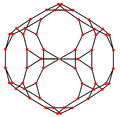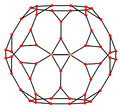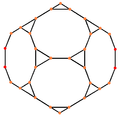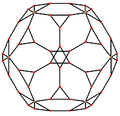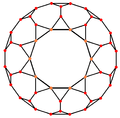Projective
symmetry
    
Dual
image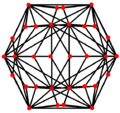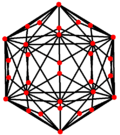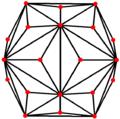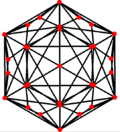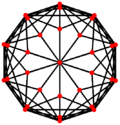## Spherical tilings and Schlegel diagrams

The truncated dodecahedron can also be represented as a spherical tiling, and projected onto the plane via a stereographic projection. This projection is conformal, preserving angles but not areas or lengths. Straight lines on the sphere are projected as circular arcs on the plane.

Schlegel diagrams are similar, with a perspective projection and straight edges.

Orthographic projection Stereographic projections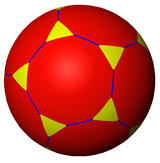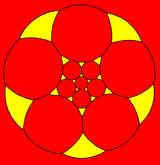Decagon-centered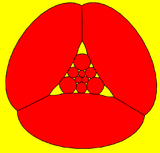Triangle-centered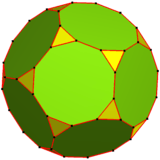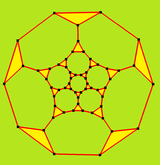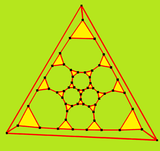## Vertex arrangement

It shares its vertex arrangement with three nonconvex uniform polyhedra:Truncated dodecahedron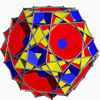Great icosicosidodecahedron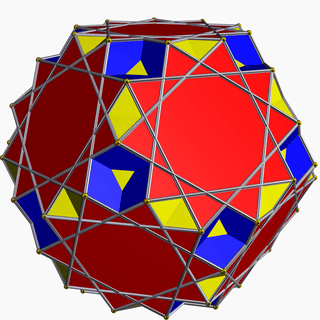Great ditrigonal dodecicosidodecahedron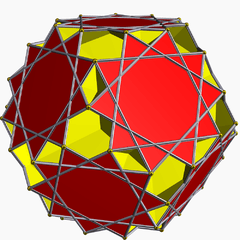Great dodecicosahedron

It is part of a truncation process between a dodecahedron and icosahedron:

This polyhedron is topologically related as a part of sequence of uniform truncated polyhedra with vertex configurations (3.2n.2n), and [n,3] Coxeter group symmetry.

## Truncated dodecahedral graph

Truncated dodecahedral graph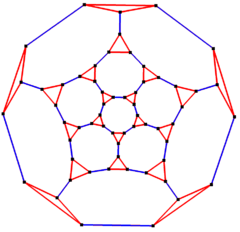5-fold symmetry schlegel diagram
Vertices 60
Edges 90
Automorphisms 120
Chromatic number 2
Properties Cubic, Hamiltonian, regular, zero-symmetric

In the mathematical field of graph theory, a truncated dodecahedral graph is the graph of vertices and edges of the truncated dodecahedron, one of the Archimedean solids. It has 60 vertices and 90 edges, and is a cubic Archimedean graph.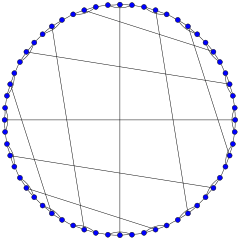Circular